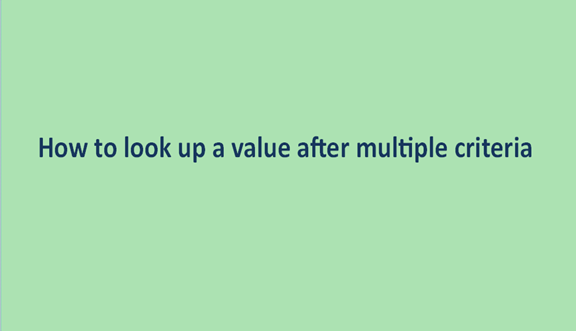## How to look up a value after multiple criteria

Looking up a value in a table using one criteria is simple. You use a simple VLOOKUP formula. Things are much different when you want to use more than one criteria- Multiple criteria. This can be attained in various ways. It includes the use of Excel functions such as LOOKUP, VLOOKUP, INDEX, and MATCH. We are going to learn from this article a few of these ways:

Using Index and MATCH function

With the help of this table:We will learn how to use the index and match functions to get the correct price for an item based on the item and the size. First, we'll look at the index and how we can use it to get the price based on one criterion.

So here we have a jacket, and we will find the price for the first Jacket.

Now we have to tell Excel which row to pull this price from. So we want to use the match function here. And it's going to find the first instance of Jacket and tell us which row that's in.

In cell D13, type =index(D2:D10,match(B13,B2:B10,0))By typing this formula match(B13, tell us that the lookup value we want is Jacket, B2:B10 defines the array in which we are to look up, and we lastly choose the type of match that we want, which is an exact match 0). This finds the value of the first Jacket.And if we look here, there's the price for the first JacketJacket. So we haven't considered the size. This is just returning that first prize. So we want to make a variation on this. We still want to look in the same range in the index, but we have to use a different method with a match to find the correct row. So we're going to be looking for a specific size when looking for the Jacket, a large-scale type.

Multiple Criteria1. Use a match to check each item and see if it's what we selected and whether it corresponds with the size. We are going to type a simple formula that compares this cell to the item name that we selected

=B2=B13 F4 to lock that reference and then copy that down.So we can see there are three truths where the JacketJacket was found.

2. Check the size. We repeat the same steps here.

Comparing the size to the selected size equals C2=C13 and press F4 and enter and then copy that down.We see three truths here at the end, and there's one spot where both are true everywhere else. It's either to falsies or at least one false.

3. The next step is to see what happens when we multiply these two columns.

=E2*F2And zeros down, except this one row where both are true. If we have to choose, we get one. So we're going to use a match that will look for one in the results.

4. Go back to the original formula retype it to

=index(D2:D10,match(1,(B13=B2:B10)*(C13=C2:C10),0))

This is an array formula. So we have to press Control, Shift, and then hit Enter. And there's our prize.